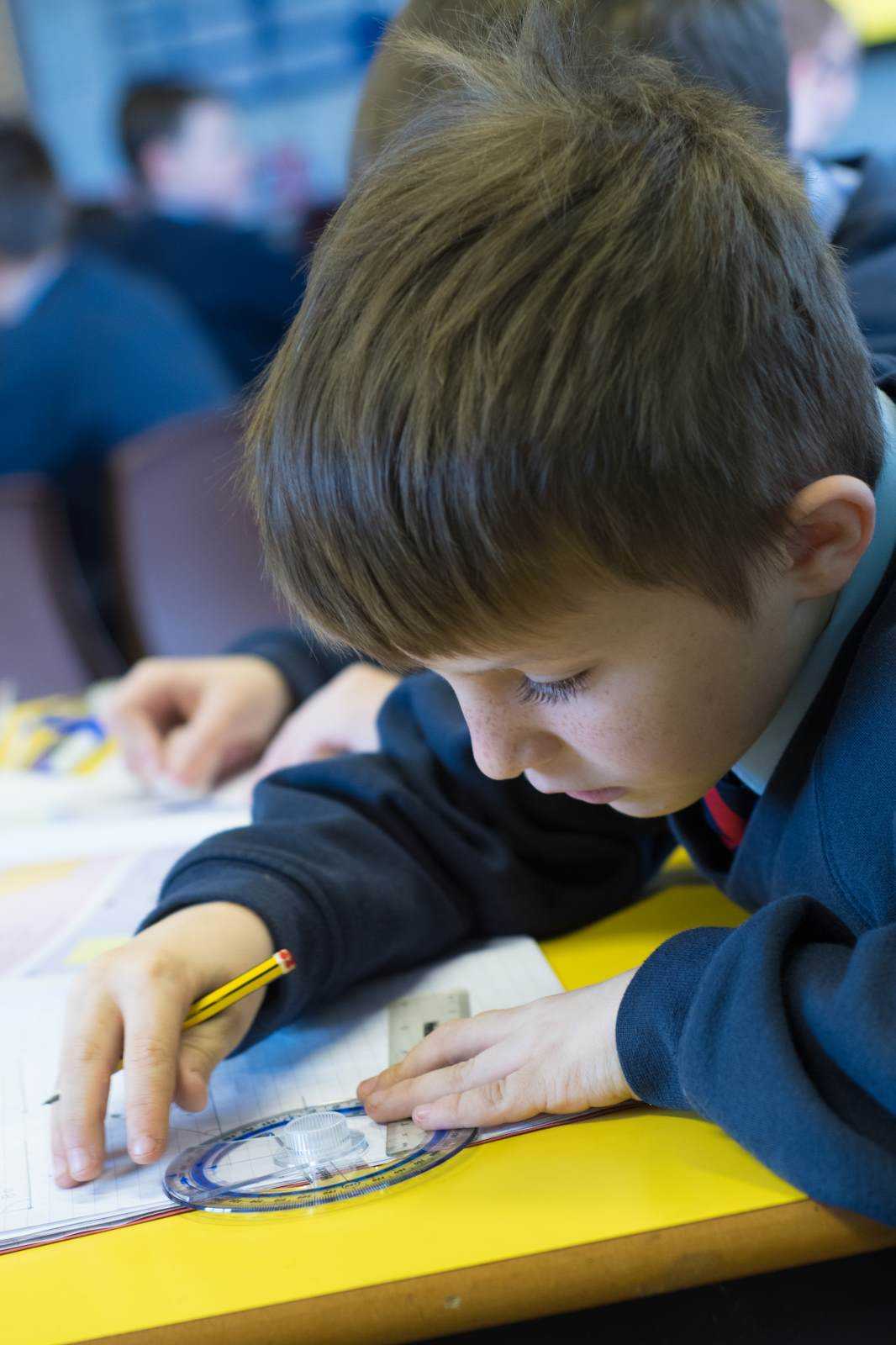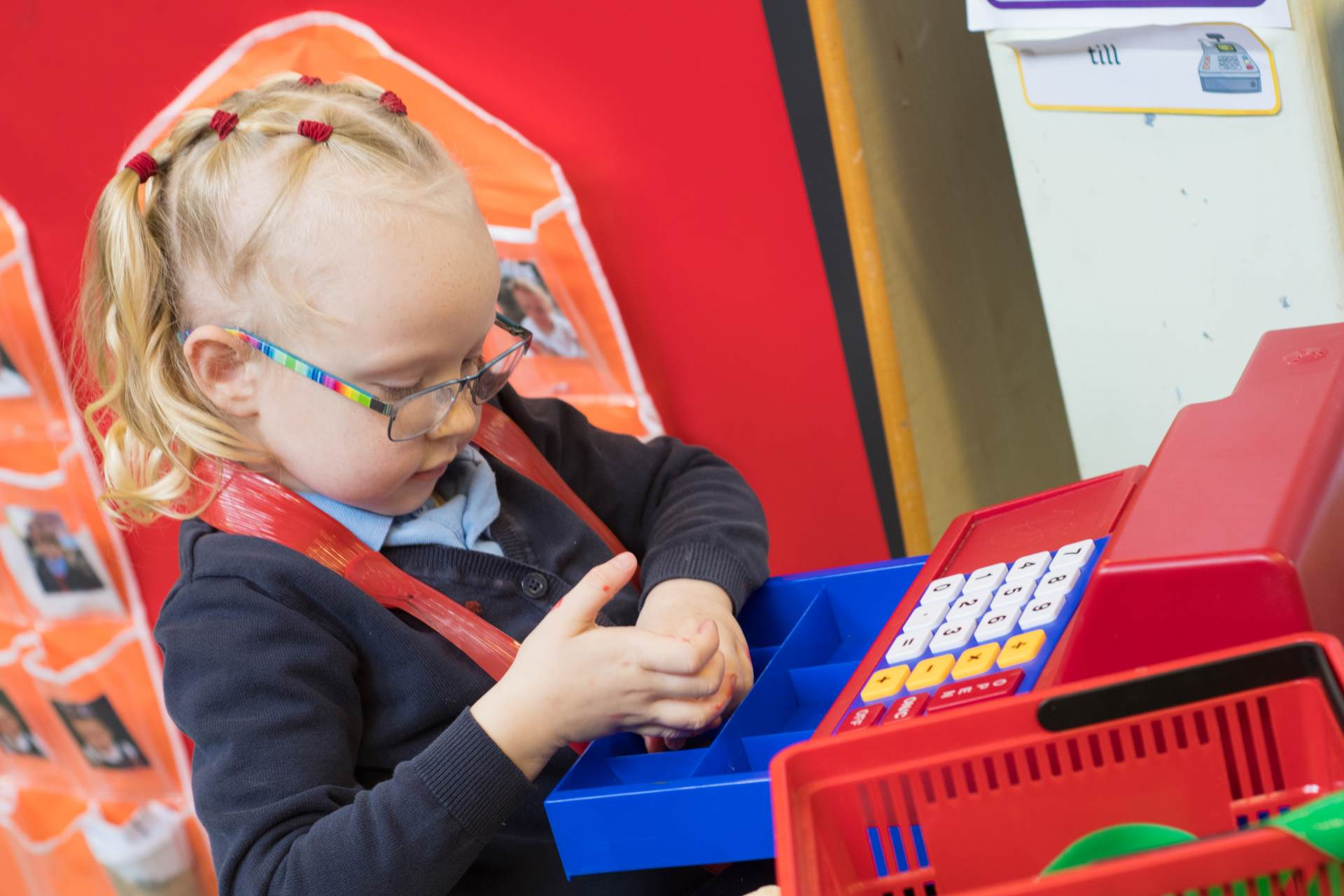MathematicsThe study of mathematics is essential to everyday life, critical to science, technology and engineering and necessary for understanding everyday finances.

At Barton Moss, we want our pupils to make rich connections across mathematical ideas to develop fluency and reasoning and to be able to solve increasingly sophisticated problems, not only in maths lessons, but in science and technology lessons and across the curriculum.

Please click on the links to find our Maths Progression documents and policies.

Year 1 Maths Curriculum

Year 2 Maths Curriculum

Year 3 Maths Curriculum

Year 4 Maths Curriculum

Year 5 Maths CurriculumYear 6 Maths Curriculum

BM Maths Policy

BM Calculation Policy

BM Bar Modelling Policy

BM Geometry Policy